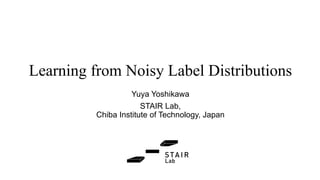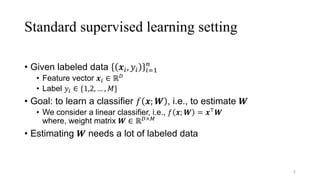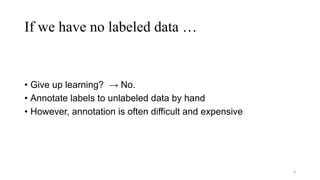Successfully reported this slideshow.

# Learning from Noisy Label Distributions (ICANN2017)×

1 of 26
1 of 26

# Learning from Noisy Label Distributions (ICANN2017)

Slides used in ICANN 2017. The paper can be downloaded from https://arxiv.org/abs/1708.04529

Slides used in ICANN 2017. The paper can be downloaded from https://arxiv.org/abs/1708.04529

### Learning from Noisy Label Distributions (ICANN2017)

1. 1. Learning from Noisy Label Distributions Yuya Yoshikawa STAIR Lab, Chiba Institute of Technology, Japan
2. 2. Standard supervised learning setting • Given labeled data 𝒙", 𝑦" "%& ' • Feature vector 𝒙" ∈ ℝ* • Label 𝑦" ∈ {1,2, … , 𝑀} • Goal: to learn a classifier 𝑓 𝒙; 𝑾 , i.e., to estimate 𝑾 • We consider a linear classifier, i.e., 𝑓 𝒙; 𝑾 = 𝒙5 𝑾 where, weight matrix 𝑾 ∈ ℝ*×7 • Estimating 𝑾 needs a lot of labeled data 2
3. 3. If we have no labeled data … • Give up learning? → No. • Annotate labels to unlabeled data by hand • However, annotation is often difficult and expensive 3
4. 4. A case that annotation is difficult • Consider annotating age (e.g., 20s, 30s, 40s) to SNS users • It’s very easy if the age is explicitly written in users’ profile • If not, annotators need to infer users’ age from: • Profile photos • Texts (tweets etc.) • Followers and followees 4 20s? 30s? difficult…
5. 5. Problem setting in this study • Goal: to learn a classifier 𝑓(𝒙, 𝑾) • Assumptions: • There is no labeled data • Each instance 𝒙" belongs to more than one groups • Each group has a noisy label distribution which can be observed • Our solution • Infer the true label distributions of the groups from the noisy ones • Infer the true label of each instance from the true label distributions • Learn a classifier 𝑓(𝒙, 𝑾) using the true labels 5
6. 6. Illustration of our setting 6
7. 7. Illustration of our setting 7 • Feature vectors 𝒙: ∈ ℝ* :%& ; for 𝑈 instances • Each instance 𝑢 has a single label 𝑦: ∈ 1, … , 𝑀 , (The shape of each instance indicates the label) • But, the label cannot be observed
8. 8. Illustration of our setting 8 • Each instance belongs to more than one groups • For each group, there is a true label distribution (unobserved)
9. 9. Illustration of our setting 9 • The true label distributions are distorted by an unknown noise • As a result, we can observe the noisy label distributions
10. 10. A typical example: Twitter 10 hyperlink Twitter world BBC News website @BBCWorld male Gender distribution of the website visitors (noisy label dist.) female 50% 50% Website world male female 60% 40% Gender distribution (true label dist.) distorted by noise
11. 11. A typical example: Twitter 11 Twitter world @BBCWorld male female 60% 40% Gender distribution (true label dist.) • Goal: to learn a classifier that predicts the gender of Twitter users • Some users follows official accounts such as @BBCWorld (BBC News) • Each user is an instance • @BBCWorld is a group • Users who follows @BBCWorld are the members of the group • Gender distribution of @BBCWorld cannot be observed
12. 12. A typical example: Twitter 12 Twitter world BBC News website @BBCWorld male Gender distribution of the website visitors (noisy label dist.) female 50% 50% Website world male female 60% 40% Gender distribution (true label dist.) distorted by noise hyperlink • @BBCWorld has a hyperlink to BBC News website • The gender distribution of the website visitors (noisy label dist.) can be obtained from audience measurement services such as Quantcast • Why is noise generated? • Twitter and website worlds have different populations • Noise is used for conforming the populations of two worlds
13. 13. Problem setting in this study • Goal: to learn a classifier 𝑓(𝒙, 𝑾) • Assumptions: • There is no labeled data • Each instance 𝒙" belongs more than one groups • Each group has a noisy label distribution which can be observed • Our solution • Infer the true label distributions of the groups from the noisy ones • Infer the true label of each instance from the true label distributions • Learn a classifier 𝑓(𝒙, 𝑾) using the inferred true labels 13
14. 14. Related work • Our study is inspired by [Cullota et al., AAAI 2015] • Our setting is almost the same as theirs • Their solution is too simple • The solution cannot capture the difference between true and noisy label distributions 14 𝒙 𝑓(𝒙, 𝑾) Training Learn a linear regression model 𝑓(𝒙, 𝑾) that predict label ratios from a feature vector 𝒙 Prediction 𝒙>?@ 𝑓(𝒙>?@, 𝑾) Return a label that have the highest label ratio predicted by 𝑓 𝒙, 𝑾 predicted ratios △ label
15. 15. Related work 15 • Our contributions • Formalized the problem by Cullota et al. as a machine learning problem • Proposed a probabilistic generative model specialized for the problem • Our study is inspired by [Cullota et al., AAAI 2015] • Our setting is almost the same as theirs • Their solution is too simple • The solution cannot capture the difference between true and noisy label distributions
16. 16. Proposed approach • Developed a probabilistic generative model that represents the generative process of the noisy label distributions 16
17. 17. Graphical model 17 Weight matrix for classifier True label of each instance Confusion matrix for noise Noisy label distributions of groups (observed) Group-dependent label for each instance and group Feature vector for each instance (observed)
18. 18. Generative process 18
19. 19. Generative process 19 𝜷 ∈ ℝ7×7 is determined by When 𝛼CD > 𝛼C& Assume strong noise When 𝛼C& > 𝛼CD Assume weak noise
20. 20. Generative process 20
21. 21. Generative process 21 𝑡:"
22. 22. Generative process 22
23. 23. Inference: variational Bayes method 23 Objective function: log of marginal posterior w.r.t. weight matrix 𝐖 and confusion matrix 𝐂 Goal: find 𝐖 and 𝐂 such that the objective function is maximized • Mean-field approximation is applied to the objective for efficient computation • Then, we estimated W and C by using a quasi-Newton method
24. 24. Experimental setting • We experimented on a synthetic dataset • The dataset is generated based on the proposed model • The purpose is to confirm that the proposed model is superior to the existing methods when the label distributions are distorted by a noise. • We created three datasets varying hyper-parameter 𝛼C& ∈ {1,10,100} • The hyper-parameter controls the strength of noise distortion • When 𝛼C&=1, noise is small, i.e., the difference between true and noisy label distributions is small • When 𝛼C&=100, noise is large, i.e., the difference between true and noisy label distributions is large 24
25. 25. Result • Regardless of noise strength, the proposed model is consistently superior to the methods proposed by [Cullota et al., AAAI 2015] 25 Table: Accuracy of true label estimation (# classes 𝑀 = 4) Methods proposed by [Cullota et al., AAAI 2015] strong noiseweak noise
26. 26. Conclusion and future work • We addressed the problem of learning a classifier from noisy label distributions • There is no labeled data • Instead, each instance belongs to more than one groups, and then, each group has a noisy label distribution • To solve this problem, we proposed a probabilistic generative model • Future work • Experiments on real-world datasets 26